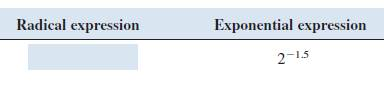# given radical expression using exponents, and given exponential expression using radicals.### Precalculus: Mathematics for Calcu...

6th Edition
Stewart + 5 others
Publisher: Cengage Learning
ISBN: 9780840068071### Precalculus: Mathematics for Calcu...

6th Edition
Stewart + 5 others
Publisher: Cengage Learning
ISBN: 9780840068071

#### Solutions

Chapter 1.2, Problem 12E
To determine

## To write: given radical expression using exponents, and given exponential expression using radicals.

Expert Solution

123 .

### Explanation of Solution

Given information:

Given expressionCalculation:

The given exponential expression is 21.5 .

Now, an=a1n and (am)n=amn

The required exponentialexpression is

21.5=232=1(23)12=123